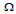# Digital Electronics - Multivibrators and 555 Timer

### Exercise :: Multivibrators and 555 Timer - General Questions

16.

Design a circuit using a 74121 to convert a 33-kHz, 30% duty cycle waveform to a 33-kHz, 60% duty cycle waveform.

 A. Rint = 2 k, Cext = 0.012 nF B. Rint = 2 k, Cext = 0.012F C. Rint = 4 k, Cext = 0.012 nF D. Rint = 4 k, Cext = 0.012F

Explanation:

No answer description available for this question. Let us discuss.

17.

What controls the output pulse width of a one shot?

 A. the clock frequency B. the width of the clock pulse C. an RL time constant D. an RC time constant

Explanation:

No answer description available for this question. Let us discuss.

18.

Does the 74123 one shot have an internal timing resistor?

 A. yes B. no

Explanation:

No answer description available for this question. Let us discuss.

19.

In a typical IC monostable multivibrator circuit, at the falling edge of the trigger input, the output switches HIGH for a period of time determined by the ________.

 A. value of the RC timing components B. amplitude of the input trigger C. frequency of the input trigger D. magnitude of the dc supply voltage

Explanation:

No answer description available for this question. Let us discuss.

20.

A monostable 555 timer has the following number of stable states:

 A. 0 B. 1 C. 2 D. 3